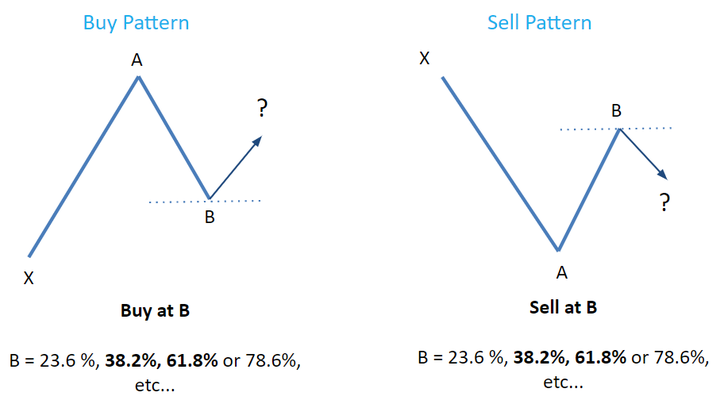# Everything You Need to Know About Fibonacci Retracement

Think back to your high school days - do you remember learning about the Fibonacci sequence? This series of numbers (0, 1, 1, 2, 3, 5, 8, etc.) is created by adding the previous two integers together. Fibonacci can be found everywhere in the world around us: from architecture and daisies to pineapples and even the spiral of a snail's shell.

One thing that high school may not have taught you, though, is that the Fibonacci sequence is also applicable to financial markets. A huge number of traders incorporate the Fibonacci sequence into their trading strategy (specifically, the "Fibonacci Retracement" technique) in order to identify the market's turning points.

Is your interest piqued? Read on to learn how you can harness the power of Fibonacci across different markets. Once you're ready to begin incorporating it into your trading strategy,  read also what is Fibonacci retracement - in it, we show you exactly how to build Fib retracement levels and use them for your success.

## The Golden Ratio

As aforementioned, the Fibonacci sequence consists of a very specific string of numbers, and it continues infinitely. There is also an associated "Golden Ratio" (also referred to as Phi or the Golden Mean). This ratio is 1.618 - and it has an inverse of 0.618. Here's how the ratio is determined:

1. When you divide an integer by the previous number in the sequence, the result will be 1.618. This figure is used in Fibonacci extensions as a key level.

2. When you divide an integer by the following number in the sequence, you will instead get 0.618. Now, this figure is how we get a 61.8% Fibonacci retracement level.

3. When dividing an integer by a number that's two ahead in the sequence, you will get 0.382 - which is where the basis for the 38.2% Fib retracement level comes from.

## Fibonacci Retracement Levels

Fibonacci retracement levels assist traders in determining support and resistance price levels in which a reversal could take place - this can be useful when trying to establish entry levels. Fib retracement levels are based on the market's previous moves. After there is a big increase in price, traders can measure the total movement from the top to the bottom - thus finding where the price might retrace to - before correcting and continuing lower.

Conversely, when there's a big decrease in price, traders will measure the fall from the top to the bottom. Here are a couple of graphs to help you visualize the pattern:Remember those important Fibonacci ratios we mentioned? Now look at the figure - you can see how the ratios of 0.618 and 0.382 are incorporated into the retracement levels. The four most commonly used Fib retracement levels are 23.6%, 38.2%, 61.8%, and 78.6%.

The "Buy at B" pattern is used when there is an uptrend in the market. Traders will try to discover how far the price retraces the X to A move (essentially, swinging low to swinging high) before they find support and bounce back higher. Those support levels are - surprise, surprise - Fibonacci retracement levels (those four percentages mentioned above).

The "Sell at B" pattern is used when there is a downtrend in the market. This time, traders will try to figure out how far the price retraces the X to A move (this one is swinging high to swinging low) before they find resistance and correct back lower). This B point could also be any of those Fibonacci retracement levels.

Traders commonly use technical analysis tools to confirm the Fibonacci retracement level that a price may turn to - such tools include price action trading patterns or trading indicators.

## Trading With Fibonacci Extension Levels

Traders can also utilize Fibonacci extension levels to calculate the distance a price might travel once the retracement is finished. In other words, Fib retracement levels are used when entering a trend, whereas Fib extension levels are used to determine where the trend will end. The Golden Ratio (1.618) forms the most commonly used Fib extension level - 161.8%.## Start Using Fibonacci Retracement

To sum up - in an uptrend, you can use Fibonacci retracement levels as a support level, in which the price can bounce and keep moving higher. Conversely, when there's a downtrend, the Fib retracement levels may act as a resistance level in which the price can bounce and correct lower. You can also determine where the trends will exit with Fibonacci extension levels.